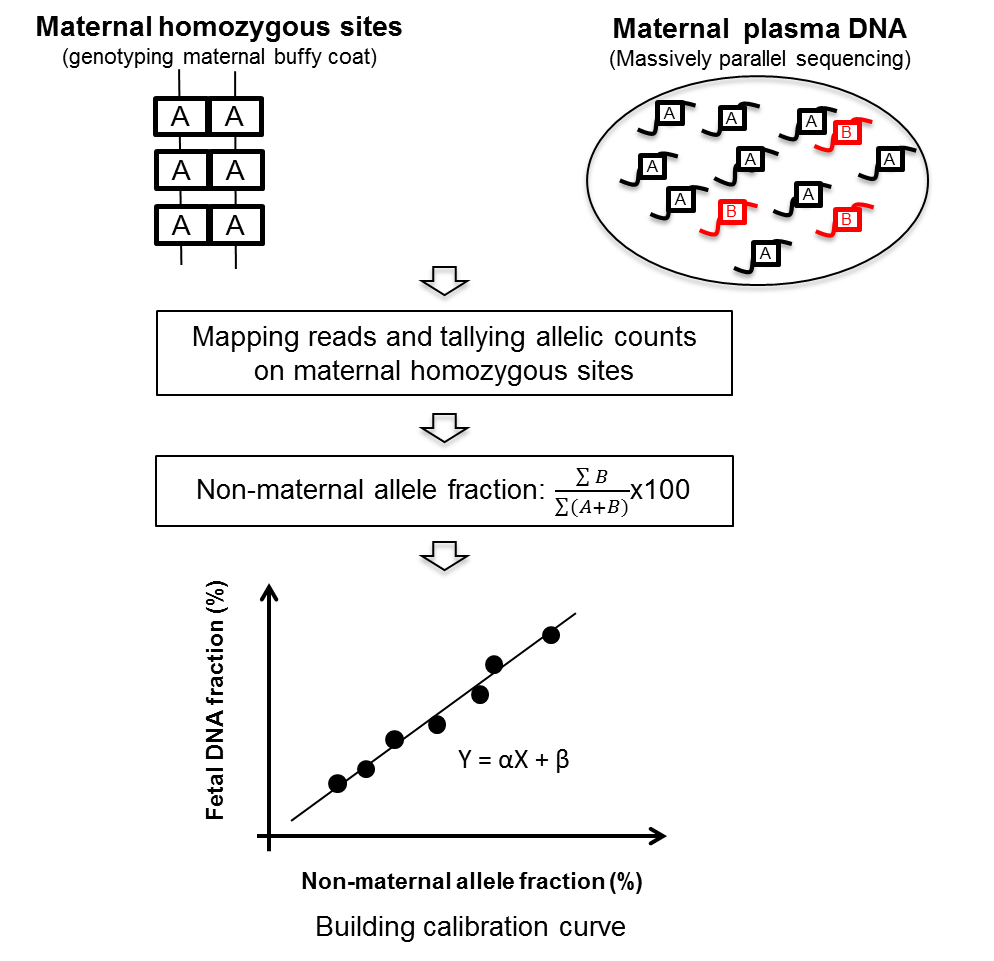# FetalQuantSD

Accurate quantification of fetal DNA fraction by shallow-depth sequencing of maternal plasma DNA

## Introduction

Noninvasive prenatal testing using massively parallel sequencing of maternal plasma DNA has been rapidly adopted in clinical use worldwide. Fetal DNA fraction in a maternal plasma sample is an important parameter for accurate interpretations of these tests. However, there is a lack of methods involving low sequencing depth and yet would allow a robust and accurate determination of fetal DNA fraction in maternal plasma for all pregnancies. In this study, we have developed a new method to accurately quantify the fetal DNA fraction by analyzing the maternal genotypes and sequencing data of maternal plasma DNA. Fetal DNA fraction was calculated based on the proportion of non-maternal alleles at single nucleotide polymorphisms (SNPs) where the mother is homozygous. This new approach achieves a median deviation of 0.6% between predicted fetal DNA fraction and the actual fetal DNA fraction using as low as 0.03 fold sequencing coverage of the human genome. We believe that this method will further enhance the clinical interpretations of noninvasive prenatal testing using genomewide random sequencing.## Manual

This pipeline is designed for the estimation of fetal DNA fraction. In the example folder, the data for a sample are included to test the programs. The file of `example/test_seq.soap` contains 1 million paired-end sequencing reads (aligned using SOAP2) and the file of `example/test_iScan.bed` contains the corresponding genotyping result (iScan platform) for this sample. Using the pipeline, the estimated fetal DNA fraction was found to be 5.26. The actual fetal DNA fraction for this sample is 5.47.

#### Step 1 Deduce linear regression model

The output file is named as `linear_parameters.dat` which contains the deduced values for slope and intercept.

`R --slave --args example/training_dataset.dat < bin/linearRegression.R`

#### Step 2 Calculate fraction of non-maternal allele

The output file is named as `test.Bfrac` which contains the value for maternal allele count, non-maternal allele count and non-maternal allele fraction.

`sh bin/FetalQuantSD.sh example/test_iScan.bed example/test_seq.soap test`

#### Step 3 Estimate fetal DNA fraction

The output value of the estimated fetal DNA fraction is printed on screen.

`perl bin/calc_fetal_frac.pl test.Bfrac linear_parameters.dat`

`tar -xvf fetalquantsd.tar.zip`